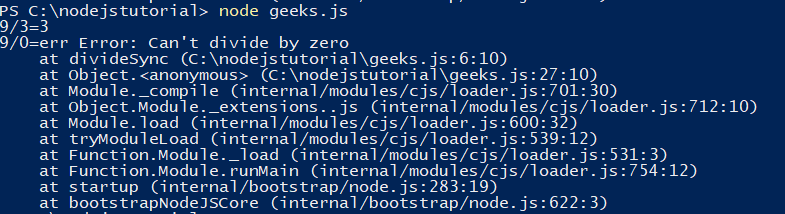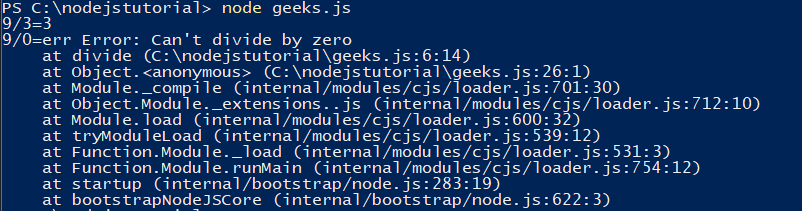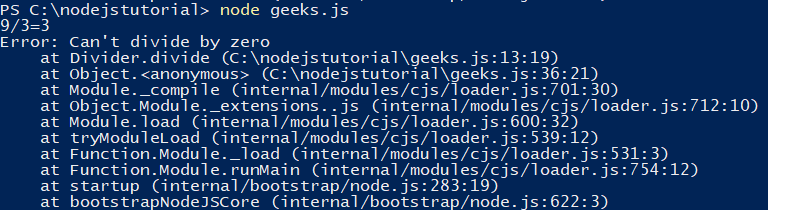# Exception Handling in Node

The exception handling refers to the mechanism by which the exceptions occurring in a code while an application is running is handled. Node.js supports several mechanisms for propagating and handling errors.
This are the different methods which can be used for exception handling in Node.js:

• Exception handling in synchronous code:If an error occurs in a synchronous code, return the error.
Example:

 `// Write Javascript code here ` `// Define divider as a syncrhonous function ` `var` `divideSync = ``function``(x, y) { ` `    ``// if error condition? ` `    ``if` `( y === 0 ) { ` `        ``// "throw" the error safely by returning it ` `        ``return` `new` `Error(``"Can't divide by zero"``) ` `    ``} ` `    ``else` `{ ` `        ``// no error occurred, continue on ` `        ``return` `x/y ` `    ``} ` `} ` ` `  `// Divide 9/3 ` `var` `result = divideSync(9, 3) ` `// did an error occur? ` `if` `( result ``instanceof` `Error ) { ` `    ``// handle the error safely ` `    ``console.log(``"9/3=err"``, result) ` `} ` `else` `{ ` `    ``// no error occurred, continue on ` `    ``console.log(``"9/3="``+result) ` `} ` ` `  `// Divide 9/0 ` `result = divideSync(9, 0) ` `// did an error occur? ` `if` `( result ``instanceof` `Error ) { ` `    ``// handle the error safely ` `    ``console.log(``"9/0=err"``, result) ` `} ` `else` `{ ` `    ``// no error occurred, continue on ` `    ``console.log(``"9/0="``+result) ` `} `

Output:• Exception handling in callback-based( asynchronous) code: In callback-based code, the one of the argument of the callback is err. If an error happens err is the error, if an error doesn’t happen then err is null. The err argument can be followed any number of other arguments.
Example:

 `// Write Javascript code here ` `var` `divide = ``function``(x, y, next) { ` `    ``// if error condition? ` `    ``if` `( y === 0 ) { ` `        ``// "throw" the error safely by calling the completion callback ` `        ``// with the first argument being the error ` `        ``next(``new` `Error(``"Can't divide by zero"``)) ` `    ``} ` `    ``else` `{ ` `        ``// no error occurred, continue on ` `        ``next(``null``, x/y) ` `    ``} ` `} ` ` `  `divide(9, 3, ``function``(err, result){ ` `    ``// did an error occur? ` `    ``if` `( err ) { ` `        ``// handle the error safely ` `        ``console.log(``"9/3=err"``, err) ` `    ``} ` `    ``else` `{ ` `        ``// no error occurred, continue on ` `        ``console.log(``"9/3="``+result) ` `    ``} ` `}) ` ` `  `divide(9, 0, ``function``(err, result){ ` `    ``// did an error occur? ` `    ``if` `( err ) { ` `        ``// handle the error safely ` `        ``console.log(``"9/0=err"``, err) ` `    ``} ` `    ``else` `{ ` `        ``// no error occurred, continue on ` `        ``console.log(``"9/0="``+result) ` `    ``} ` `}) `

Output:• Exception handling in eventful code:In an eventful code, the error may happen anywhere. So instead of throwing the error, fire the error event instead.
Example:

 `// Write Javascript code here ` `// Definite our Divider Event Emitter ` `var` `events = require(``"events"``) ` `var` `Divider = ``function``(){ ` `    ``events.EventEmitter.call(``this``) ` `} ` `require(``'util'``).inherits(Divider, events.EventEmitter) ` ` `  `// Add the divide function ` `Divider.prototype.divide = ``function``(x, y){ ` `    ``// if error condition? ` `    ``if` `( y === 0 ) { ` `        ``// "throw" the error safely by emitting it ` `        ``var` `err = ``new` `Error(``"Can't divide by zero"``) ` `        ``this``.emit(``"error"``, err) ` `    ``} ` `    ``else` `{ ` `        ``// no error occurred, continue on ` `        ``this``.emit(``"divided"``, x, y, x/y) ` `    ``} ` ` `  `    ``// Chain ` `    ``return` `this``; ` `} ` ` `  `// Create our divider and listen for errors ` `var` `divider = ``new` `Divider() ` `divider.on('error``', function(err){ ` `    ``// handle the error safely ` `    ``console.log(err) ` `}) ` `divider.on('``divided', ``function``(x, y, result){ ` `    ``console.log(x+``"/"``+y+``"="``+result) ` `}) ` ` `  `// Divide ` `divider.divide(9, 3).divide(9, 0) `

Output:My Personal Notes arrow_drop_upCheck out this Author's contributed articles.

If you like GeeksforGeeks and would like to contribute, you can also write an article using contribute.geeksforgeeks.org or mail your article to contribute@geeksforgeeks.org. See your article appearing on the GeeksforGeeks main page and help other Geeks.

Please Improve this article if you find anything incorrect by clicking on the "Improve Article" button below.

Improved By : Akanksha_Rai

Article Tags :

Be the First to upvote.

Please write to us at contribute@geeksforgeeks.org to report any issue with the above content.